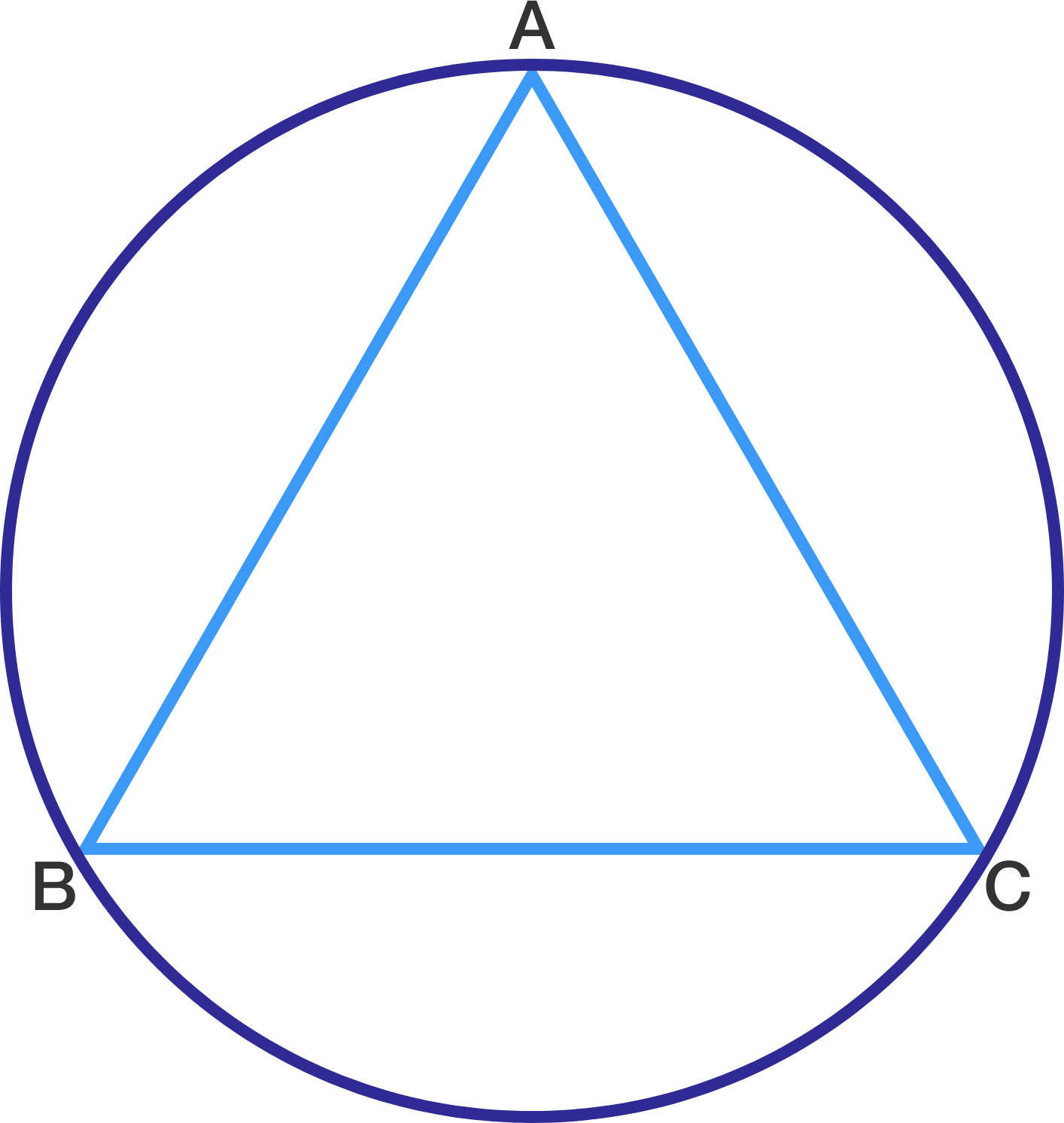# Triangle in circle

Geometry Level 1In the figure, $\triangle ABC$ is an equilateral triangle where $AB = 6$ cm. The area of the circumcircle is $k\pi$ cm$^{2}$. Find the value of $k$.

×

Problem Loading...

Note Loading...

Set Loading...